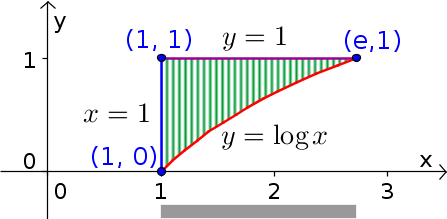# Math Insight

### Image: Change order of integration example region with exponential, y firstExample region illustrating process of changing the order of integration in double integrals, shown with y as the inner integral. For a given value of $x$, $y$ ranges from the curve $y=\log x$ (red) to the line $y=1$ (purple), as illustrated by the vertical hashing over the region. The total range of $x$ over the region is from 1 to $e$, as illustrated by the gray bar at the bottom.

Source image file: double_integral_change_order_example_exp_y.ggb
Source image type: Geogebra